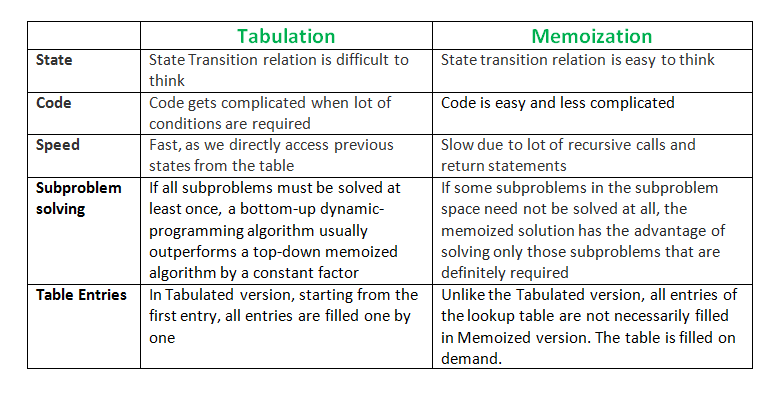Tabulation vs Memoization

• Difficulty Level : Easy
• Last Updated : 30 Dec, 2021

Prerequisite – Dynamic Programming, How to solve Dynamic Programming problems?
There are two different ways to store the values so that the values of a sub-problem can be reused. Here, will discuss two patterns of solving dynamic programming (DP) problems:

1. Tabulation: Bottom Up
2. Memoization: Top Down

Before getting to the definitions of the above two terms consider the following statements:

• Version 1: I will study the theory of DP from GeeksforGeeks, then I will practice some problems on classic DP and hence I will master DP.
• Version 2: To Master DP, I would have to practice Dynamic problems and practice problems – Firstly, I would have to study some theories of DP from GeeksforGeeks

Both versions say the same thing, the difference simply lies in the way of conveying the message and that’s exactly what Bottom-Up and Top-Down DP do. Version 1 can be related to Bottom-Up DP and Version-2 can be related as Top-Down DP.

Tabulation Method – Bottom Up Dynamic Programming

As the name itself suggests starting from the bottom and accumulating answers to the top. Let’s discuss in terms of state transition.

Let’s describe a state for our DP problem to be dp[x] with dp as base state and dp[n] as our destination state. So,  we need to find the value of destination state i.e dp[n].
If we start our transition from our base state i.e dp and follow our state transition relation to reach our destination state dp[n], we call it the Bottom-Up approach as it is quite clear that we started our transition from the bottom base state and reached the topmost desired state.

Now, Why do we call it the tabulation method?

To know this let’s first write some code to calculate the factorial of a number using a bottom-up approach. Once, again as our general procedure to solve a DP we first define a state. In this case, we define a state as dp[x], where dp[x] is to find the factorial of x.

Now, it is quite obvious that dp[x+1] = dp[x] * (x+1)

// Tabulated version to find factorial x.
int dp[MAXN];

// base case
int dp = 1;
for (int i = 1; i< =n; i++)
{
dp[i] = dp[i-1] * i;
}

The above code clearly follows the bottom-up approach as it starts its transition from the bottom-most base case dp and reaches its destination state dp[n]. Here, we may notice that the DP table is being populated sequentially and we are directly accessing the calculated states from the table itself and hence, we call it the tabulation method.

Memoization Method – Top-Down Dynamic Programming

Once, again let’s describe it in terms of state transition. If we need to find the value for some state say dp[n] and instead of starting from the base state that i.e dp we ask our answer from the states that can reach the destination state dp[n] following the state transition relation, then it is the top-down fashion of DP.

Here, we start our journey from the top most destination state and compute its answer by taking in count the values of states that can reach the destination state, till we reach the bottom-most base state.

Once again, let’s write the code for the factorial problem in the top-down fashion

// Memoized version to find factorial x.
// To speed up we store the values
// of calculated states

// initialized to -1
int dp[MAXN]

// return fact x!
int solve(int x)
{
if (x==0)
return 1;
if (dp[x]!=-1)
return dp[x];
return (dp[x] = x * solve(x-1));
}

As we can see we are storing the most recent cache up to a limit so that if next time we got a call from the same state we simply return it from the memory. So, this is why we call it memoization as we are storing the most recent state values.

In this case, the memory layout is linear that’s why it may seem that the memory is being filled in a sequential manner like the tabulation method, but you may consider any other top-down DP having 2D memory layout like Min Cost Path, here the memory is not filled in a sequential manner.This article is contributed by Nitish Kumar. If you like GeeksforGeeks and would like to contribute, you can also write an article using or mail your article to review-team@geeksforgeeks.org. See your article appearing on the GeeksforGeeks main page and help other Geeks.